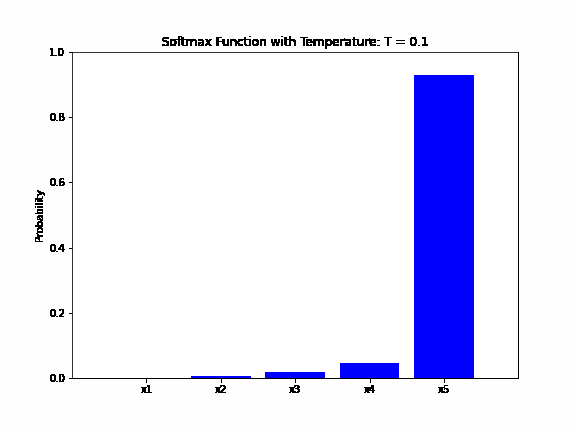## 1. Introduction

This short article’ll explain the softmax function and its relationship with temperature. Also, we’ll discuss the reasons behind incorporating temperature into the softmax function.

## 2. Softmax

The softmax function is an activation function often used as an output function in the last layer of a neural network. This function is a generalization of the logistic function for more than two variables.

Softmax takes a vector of real numbers as input and normalizes it into a probability distribution. The output of the softmax function is a vector of the same dimension as the input, with each element in the range of 0 to 1. Also, the sum of all elements are equal to 1.

Mathematically, we define the softmax function as:

(1)whereis an input vector and valuesare in range fromto.

## 3. Temperature in Softmax

The term “softmax” comes from the words “soft” and “max”. The “soft” part indicates that the function produces a probabilistic distribution that is softer than a hard maximum function. The “max” part means that it will select the maximum value in the input vector as the most likely choice but in a soft, probabilistic manner.

For example, if we have an input vector of, the hard maximum function will output a vector of. In contrast, the output of the softmax function will be.

The temperature parameter is introduced in a softmax function to control the “softness” or “peakiness” of the output probability distribution. The temperature is a parameter that we use to control the level of randomness in the function’s output. Mathematically, a softmax function with the temperature parameter T can be defined as:

(2)The temperature parametercan take on any numerical value. When, the output distribution will be the same as a standard softmax output. The higher the value of, the “softer” the output distribution will become. For example, if we wish to increase the randomness of the output distribution, we can increase the value of the parameter.

The animation below shows how the output probability of the softmax function changes as the temperature parameter varies. The input vector isand the temperature changes fromtowith a step of:## 4. Why Use Temperature in Softmax

The temperature can be useful in cases when we want to introduce more randomness or diversity in the output distribution. This is especially useful in language models for text generating, where the output distribution represents the probability of the next word token. If our model is often overconfident, it may produce very repetitive text.

For example, the temperature is a hyperparameter used in language models such as GPT-2, GPT-3, and BERT to control the randomness of the generated text. The current version of ChatGPT (gpt-3.5-turbo model) also uses temperature with softmax function.

ChatGPT has a vocabulary of 175,000 subwords, which is the same as the number of dimensions in the input and output vectors of the softmax function. Each dimension in the output of the softmax function corresponds to the probability of a particular word in the vocabulary being the next word in the sequence. Therefore, ChatGPT API has a temperature parameter that can take values between 0 and 2 to control randomness and creativity in the generated text. The default value is 1.

## 5. Conclusion

In this article, we’ve explained the softmax function with temperature and why to use it. We have also mentioned some applications where softmax with temperature is used.